# The Arrows of Time

post by lsusr · 2019-12-21T11:42:16.894Z · score: 5 (3 votes) · LW · GW · 6 comments

## Contents

  Relativistic Time
Entropic Time
None


Imagine two rocks in one-dimensional newtonian space. They come at each other, collide elastically and then bounce away. We can draw the situation with time on the vertical axis and space on the horizontal axis.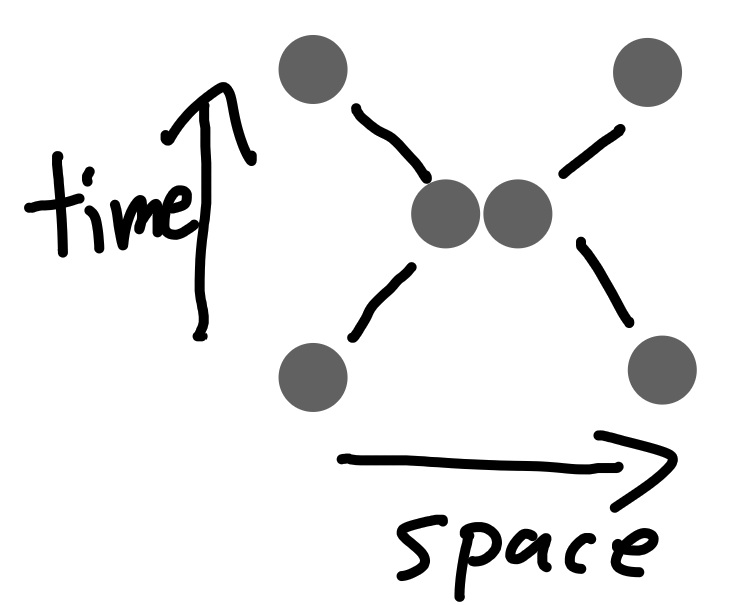We don't have to point the arrow of time straight up. We can rotate it up to 45 degrees clockwise of counterclockwise and newtonian mechanics still works.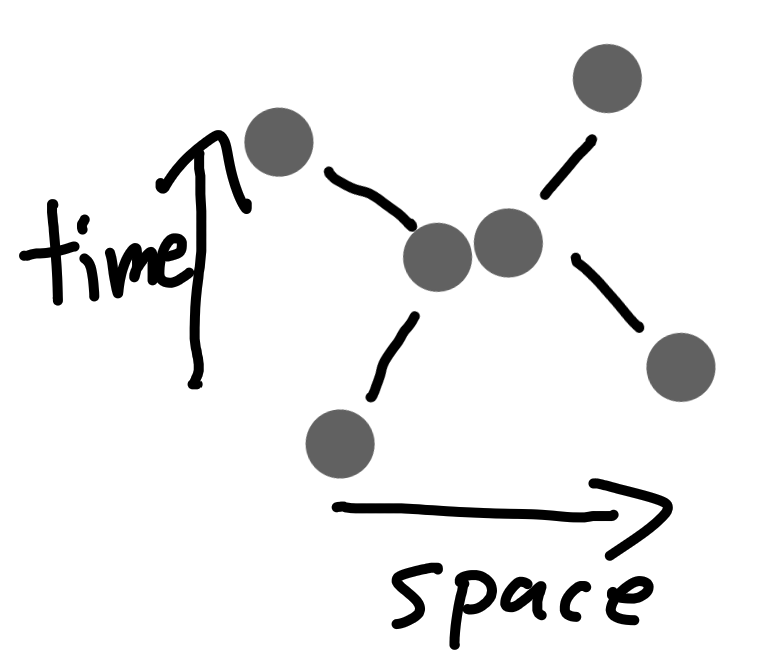We can even reverse it.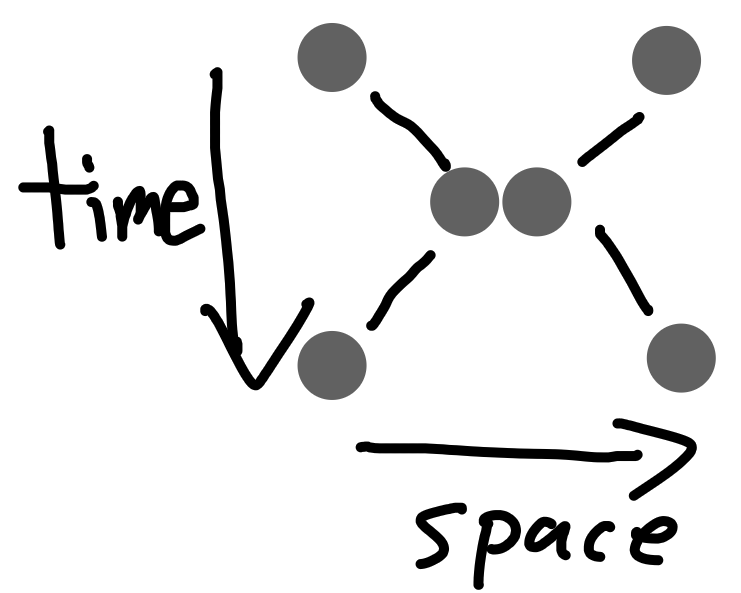We can point the arrow of time many different directions. Half of the unit circle is valid.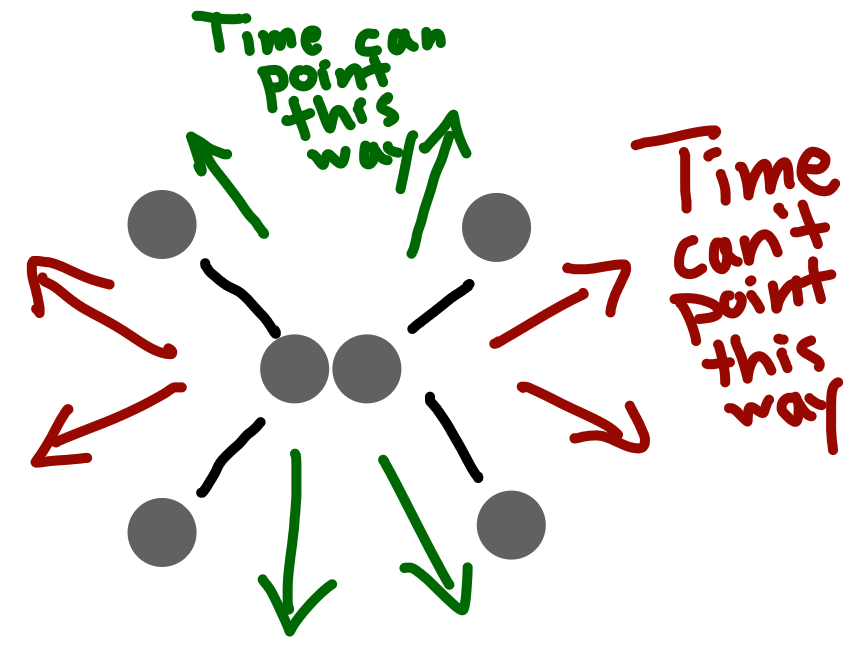But the real world has more than two rocks in it. Suppose we had multiple collisions at different angles. We must point the arrow of time in a direction that is allowed by all collisions. The only direction allowed by every possible collision is straight up, right?

# Relativistic Time

Wrong. When we're playing with spacetime like this we need to use special relativity instead of classical relativity. In special relativity, space and time have the same units where one second of time (one second) equals one light-second of space ( meters).

Our two rocks can't be travelling at 45 degrees because that's the speed of light and matter can't move at the speed of light. Instead, the rocks must be travelling steeper angles in our diagram.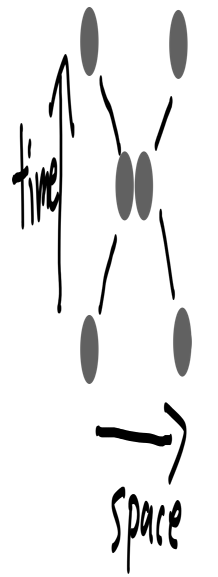Applying special relativity also means we can't literally rotate our image a certain number of degrees. What we were previously calling a "rotation" is more robustly defined as a "change in reference frame". Instead of literally rotating the diagram we have to perform a Lorentz transformation. With Lorentz transformations the same 180 degrees are valid for all collisions. Everything works out. This solves the problem of multiple collisions at different angles.

We've now proved that time can point in an infinite number of different directions. In the real world time feels like it goes one direction. How do we know which direction this is in our universe of floating rocks?

It depends on your perspective. If you're one of the rocks then your perception of time is perpendicular to your trajectory in space. Your spacetime trajectory is a line. It's possible to follow this line in two directions. We still must figure out which end is forward in time and which one is backward in time.

The past is distinguished from the future via differences in entropy. One direction has greater entropy than the other. The direction toward increasing entropy is the future and the direction towards decreasing entropy is the past.

The region of allowable time arrows is separated into two non-adjacent regions separated by the region of disallowed time arrows. One of the two allowable regions has higher entropy than the other. So "future" refers to the set of green arrows with higher entropy and "past" refers to set of green arrows with lower entropy.

# Entropic Time

"Entropy" means multiplicy of possible microstates for a particular macrostate.

Suppose a universe is made up of quantum particles each of which has two states: 0 and 1. In this sense, the entire universe is just a number like 0b0000000000000000. Our microstate is the entire 16-digit binary number. Our macrostate is the total number of 1s in the string. I will use to denote the macrostate with particles in the 1 state. So the macrostate corresponding to microstate 0b0001001001001000 is because there are 4 ones in the binary microstate.

Let's suppose the lifetime of a quantum particle is 1/16 instants. To simplify the math let's also assume that every instant exactly one quantum particle changes its state.

Our universe now consists of possible states. We can plot them out in a 16-dimensional hypercube. Every point in the hypercube is connected to exactly 16 other universe microstates. Every edge corresponds to a possible past and future of each instant. From this perspective there is no arrow of time. To a microstate, each of the 16 adjacent microstates is equally likely.

That was looking at the universe in terms of microscates. If we look at the universe in terms of macrostates then we get a one-dimensional line from to . Each macrostate can change into a macrostate one greater or one lesser than itself. (Except and which must move to and , respectively.) So the macrostate can become or in the next instant. But they're not equally likely. The macrostate has microstates. The macrostate has microstates so the macrostate has a 97% chance of entering the macrostate and a 3% chance of entering the macrostate. That's another way of saying the macrostate has greater entropy than the macrostate.

The heat death of our universe is macrostate , which has maximum entropy. But there are two beginnings of minimal entropy: and . If the real world is like this then there could be another big bang (or more) on the other side of the heat death of the universe.

1. Though this works fine as a first-order approximation at non-relativistic speeds. ↩︎

2. The prefix "" indicates when a number is written in binary (base 2). ↩︎

3. I wonder if this could be related to Baryon asymmetry. ↩︎

comment by shminux · 2019-12-21T19:49:33.363Z · score: 4 (6 votes) · LW(p) · GW(p)

Idle speculating is fun and all, but consider reading an academic book or two on the topic. Then learn and do the math. I could point out the errors in the above, like confusing classical and quantum bits, but it's best if you do it yourself some day.

comment by lsusr · 2019-12-21T21:23:05.158Z · score: 5 (5 votes) · LW(p) · GW(p)

I could point out the errors in the above, like confusing classical and quantum bits...

Could you point out the errors please?

It's not obvious to me why the difference between classical and quantum bits matters in this thought experiment because superposition and entanglement are not supposed to be part of it. I did admittedly gloss over how a quantum bit needs to be measured to force it into one of two states. Does that really invalidate the thought experiment?

[C]onsider reading an academic book or two on the topic. Then learn and do the math...it's best if you do it yourself some day.

I read several academic books on physics and math when I got my bachelor's degree in physics and math. I drilled freshman-level special relativity and quantum mechanics many times when I tutored these subjects. The footnote on Baryon asymmetry is indeed wild speculation, but the rest of the microstate/macrostate stuff generalizes from a statistical mechanics class I took in my sophomore year of college. Which additional math do you recommend I learn how to do?

comment by shminux · 2020-01-14T03:44:49.969Z · score: 2 (1 votes) · LW(p) · GW(p)
Suppose a universe is made up of 16 quantum particles each of which has two states: 0 and 1. In this sense, the entire universe is just a number like 0b0000000000000000.

Well, if your universe is just two states, its description in the eigenstate basis would be something like A1 exp(iE1 t)|1> + A2 exp(iE2 t), where A1 and A2 are complex and E1 and E2 are real (modulo normalization and phase). I am not sure how this maps into a finite length binary number.

comment by lsusr · 2020-01-20T07:29:12.402Z · score: 1 (1 votes) · LW(p) · GW(p)

It maps to a finite length binary number if you force the particle into one of two states. So you could think of this universe as equidistant (in time) instants of a continuous universe where positions are measured, then they're let to evolve and then positions are measured again. The binary strings refer only to the snapshots where the continuous universe is measured.

This ignores the fact that there must be something to measure the particles with. The goal of this thought experiment is to play around with the Born rule [LW · GW] while ignoring the time evolution of a wave function governed by the Schrödinger equation.

comment by sflicht · 2020-01-05T02:17:32.009Z · score: 2 (2 votes) · LW(p) · GW(p)

2^16 != 1632

comment by lsusr · 2020-01-05T02:22:05.381Z · score: 1 (1 votes) · LW(p) · GW(p)

Fixed. Thanks.